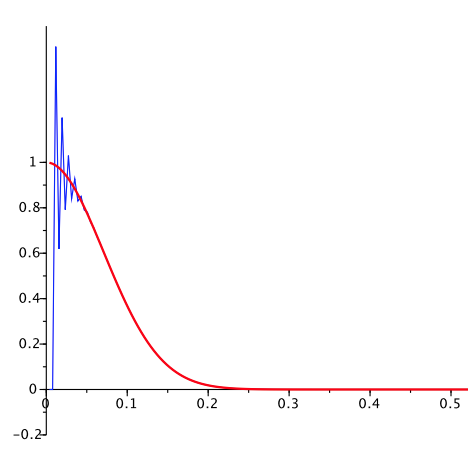# Stability of finite difference schemes for hyperbolic initial boundary value problems: numerical boundary layers### Résumé

In this article, we give a unified theory for constructing boundary layer expansions for discretized transport equations with homogeneous Dirichlet boundary conditions. We exhibit a natural assumption on the discretization under which the numerical solution can be written approximately as a two-scale boundary layer expansion. In particular, this expansion yields discrete semigroup estimates that are compatible with the continuous semigroup estimates in the limit where the space and time steps tend to zero. The novelty of our approach is to cover numerical schemes with arbitrarily many time levels.

Type
Publication
Numerical Mathematics: Theory, Methods and Applications